# SUBTOPIC Charge units Electric field Electric force Coulombs

• Slides: 60SUBTOPIC • Charge units • Electric field • Electric force & Coulomb’s Law • Capacitance and unit • Parallel plate capacitor • Dielectric constant and it’s function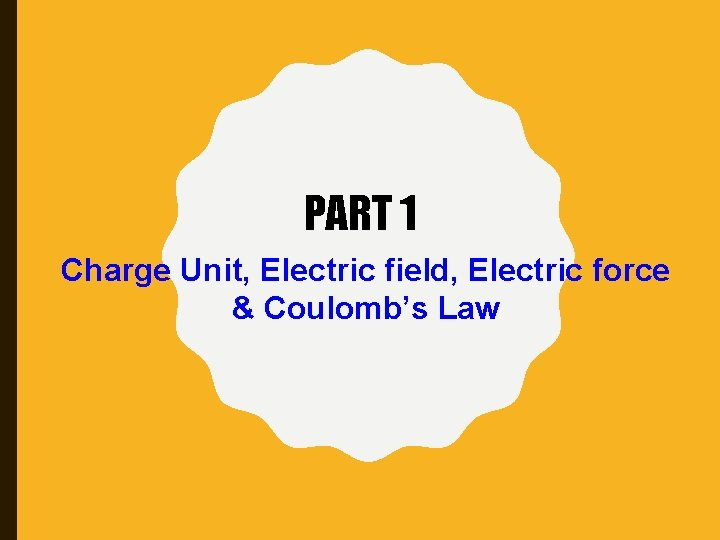PART 1 Charge Unit, Electric field, Electric force & Coulomb’s Law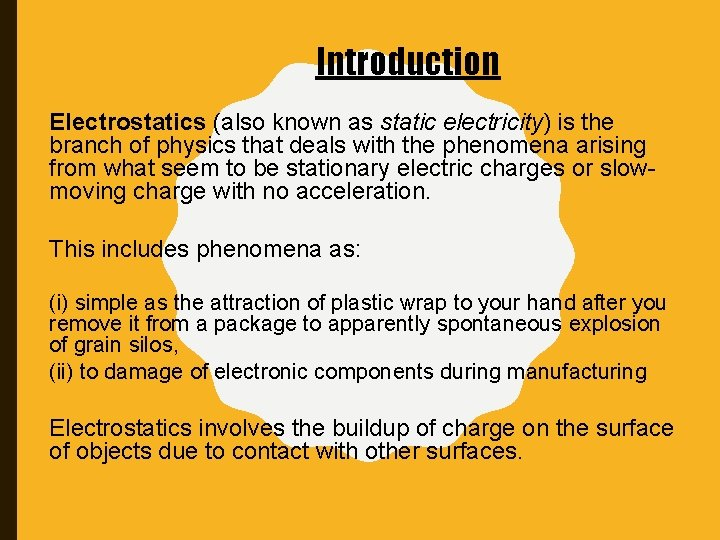Introduction Electrostatics (also known as static electricity) is the branch of physics that deals with the phenomena arising from what seem to be stationary electric charges or slowmoving charge with no acceleration. This includes phenomena as: (i) simple as the attraction of plastic wrap to your hand after you remove it from a package to apparently spontaneous explosion of grain silos, (ii) to damage of electronic components during manufacturing Electrostatics involves the buildup of charge on the surface of objects due to contact with other surfaces.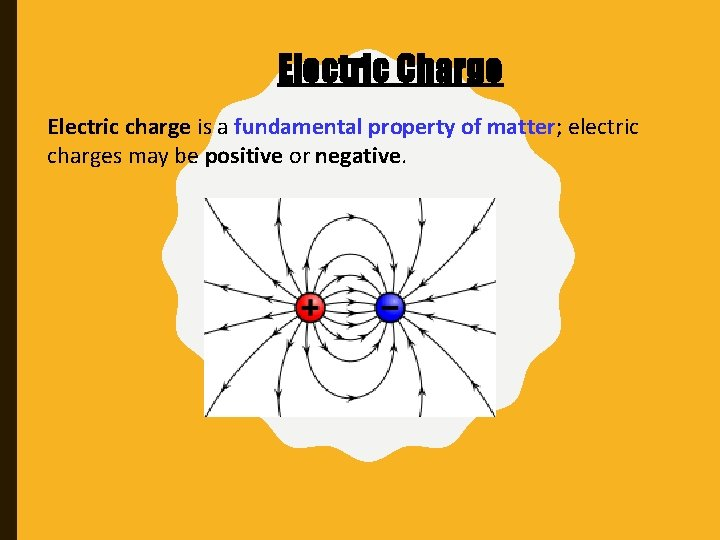Electric Charge Electric charge is a fundamental property of matter; electric charges may be positive or negative.THE ATOM CONSISTS OF A SMALL POSITIVE NUCLEUS IS SURROUNDED BY A NEGATIVE ELECTRON CLOUD.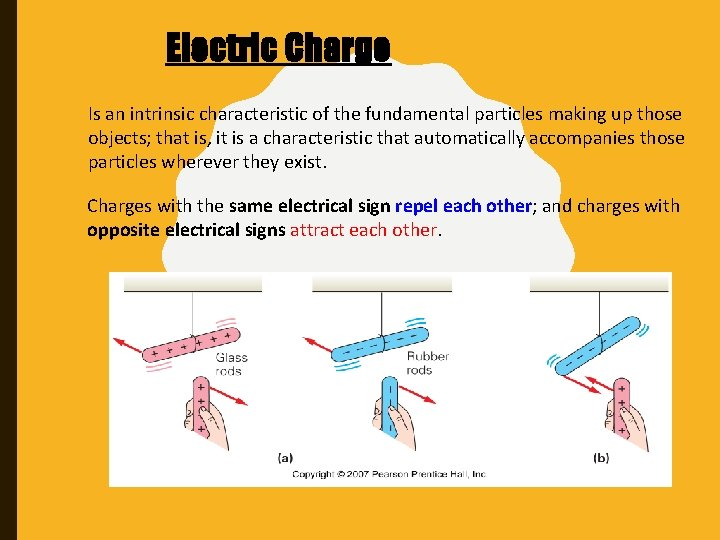Electric Charge Is an intrinsic characteristic of the fundamental particles making up those objects; that is, it is a characteristic that automatically accompanies those particles wherever they exist. Charges with the same electrical sign repel each other; and charges with opposite electrical signs attract each other.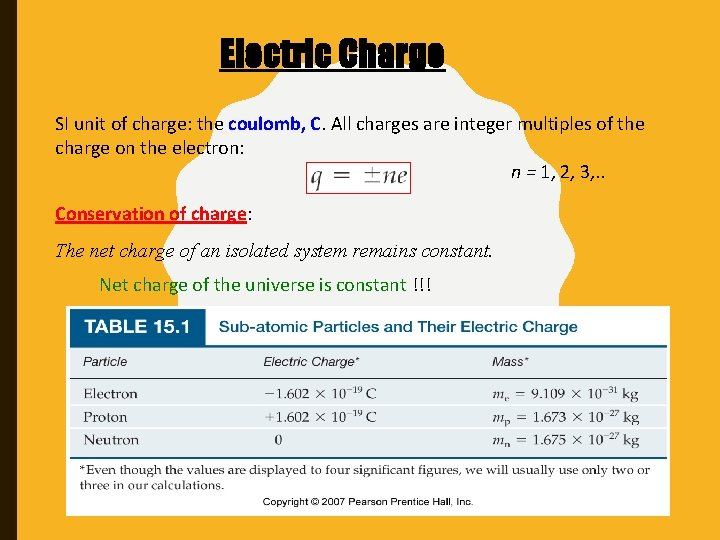Electric Charge SI unit of charge: the coulomb, C. All charges are integer multiples of the charge on the electron: n = 1, 2, 3, . . Conservation of charge: The net charge of an isolated system remains constant. Net charge of the universe is constant !!!Electrostatic Charging Conductors transmit charges readily. Semiconductors are intermediate; their conductivity can depend on impurities and can be manipulated by external voltages. Insulators do not transmit charge at all.Electrostatic Charging An electroscope may be used to determine if an object is electrically charged.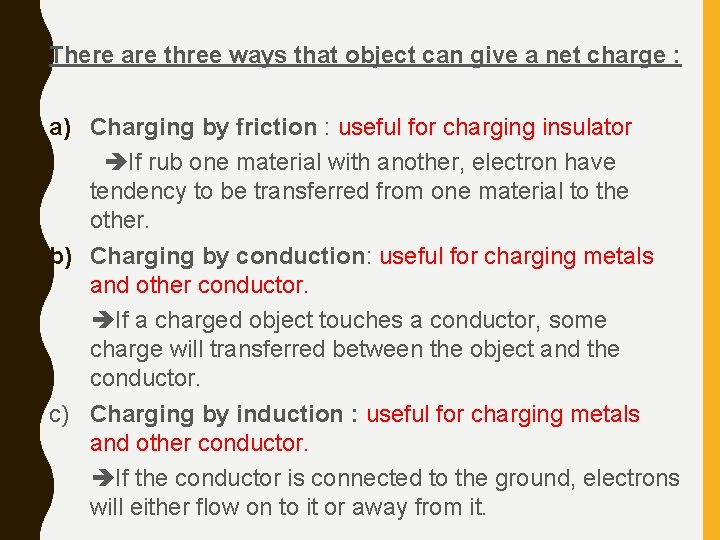There are three ways that object can give a net charge : a) Charging by friction : useful for charging insulator If rub one material with another, electron have tendency to be transferred from one material to the other. b) Charging by conduction: useful for charging metals and other conductor. If a charged object touches a conductor, some charge will transferred between the object and the conductor. c) Charging by induction : useful for charging metals and other conductor. If the conductor is connected to the ground, electrons will either flow on to it or away from it.Electrostatic Charging: FRICTION Charging by friction: This is the process by which you get “charged up” walking across the carpet in the winter. It is also the process that creates “static cling” in your laundry, and makes it possible for you to rub a balloon on your hair and then stick the balloon to the wall.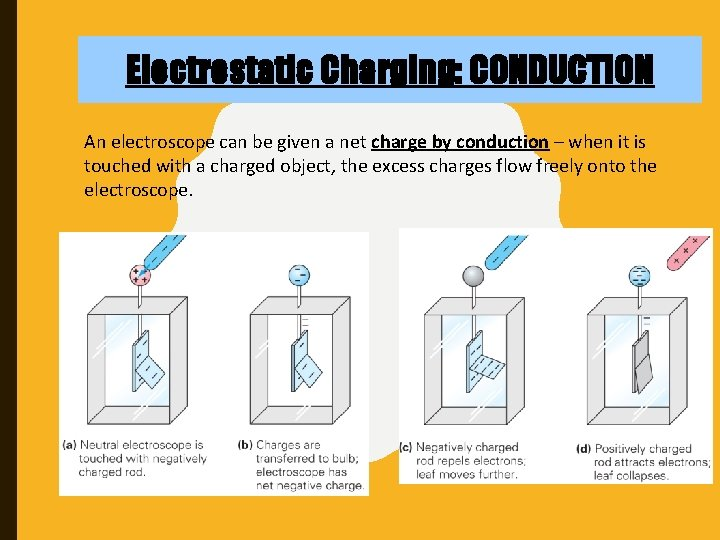Electrostatic Charging: CONDUCTION An electroscope can be given a net charge by conduction – when it is touched with a charged object, the excess charges flow freely onto the electroscope.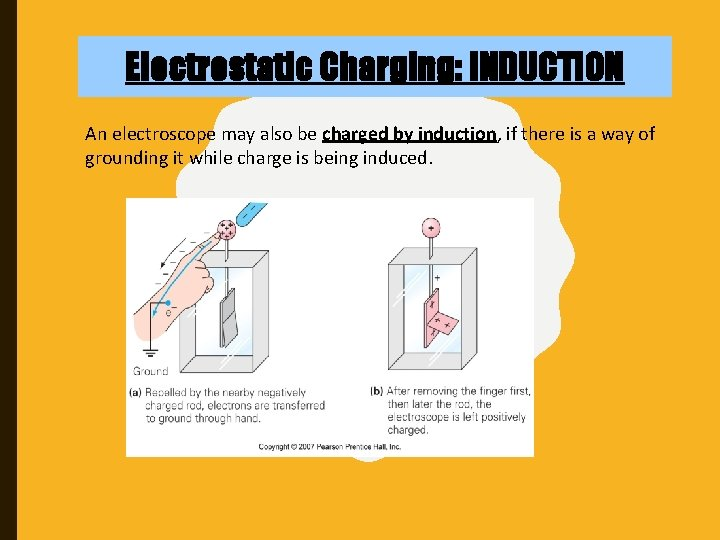Electrostatic Charging: INDUCTION An electroscope may also be charged by induction, if there is a way of grounding it while charge is being induced.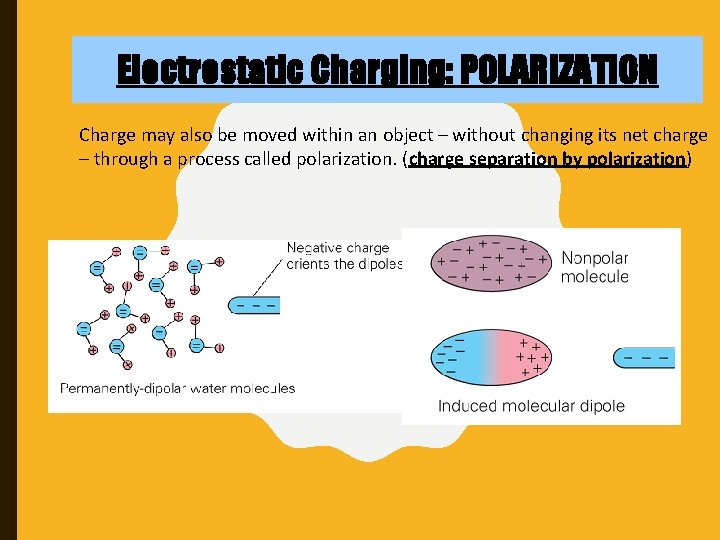Electrostatic Charging: POLARIZATION Charge may also be moved within an object – without changing its net charge – through a process called polarization. (charge separation by polarization)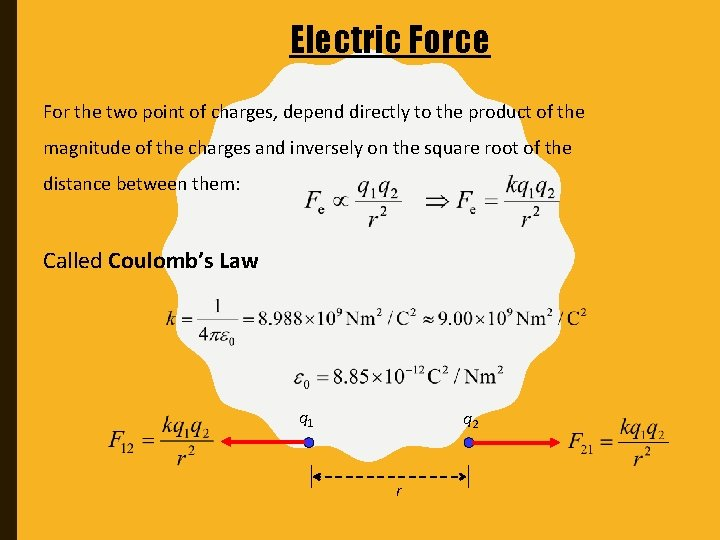Electric Force For the two point of charges, depend directly to the product of the magnitude of the charges and inversely on the square root of the distance between them: Called Coulomb’s Law q 1 q 2 r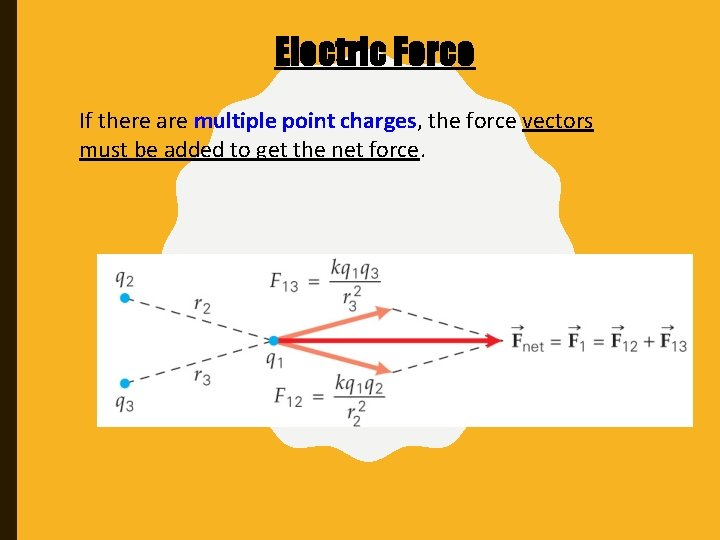Electric Force If there are multiple point charges, the force vectors must be added to get the net force.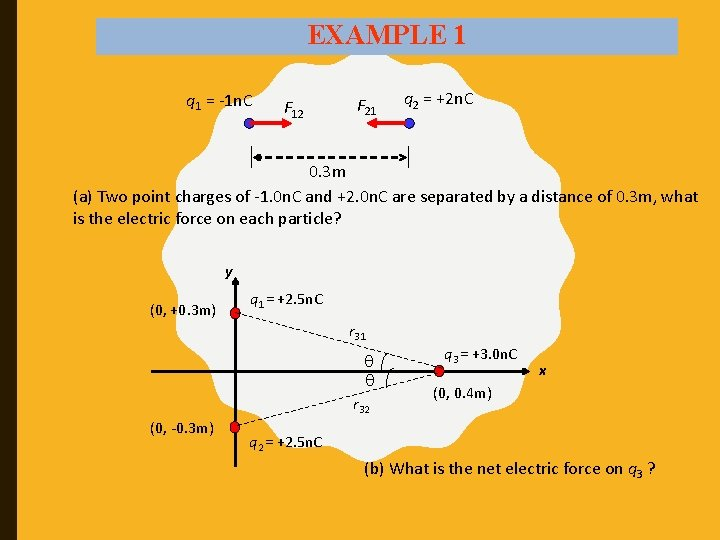EXAMPLE 1 q 1 = -1 n. C F 12 F 21 q 2 = +2 n. C 0. 3 m (a) Two point charges of -1. 0 n. C and +2. 0 n. C are separated by a distance of 0. 3 m, what is the electric force on each particle? y (0, +0. 3 m) q 1 = +2. 5 n. C r 31 r 32 (0, -0. 3 m) q 3 = +3. 0 n. C x (0, 0. 4 m) q 2 = +2. 5 n. C (b) What is the net electric force on q 3 ?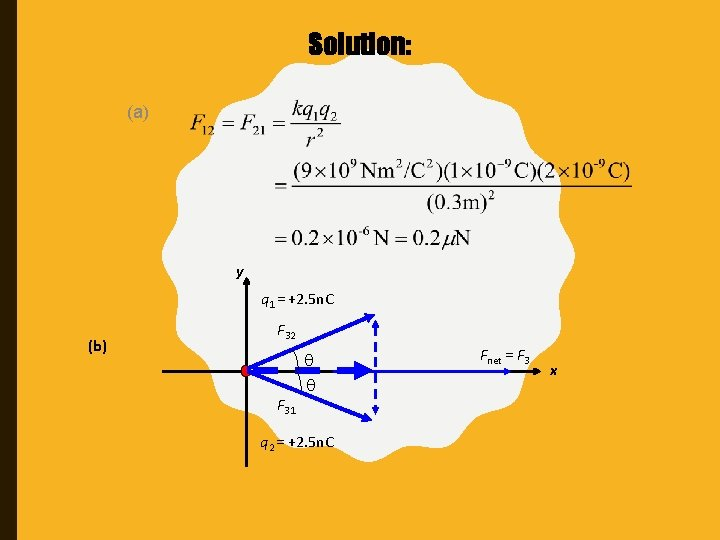Solution: (a) y q 1 = +2. 5 n. C (b) F 32 F 31 q 2 = +2. 5 n. C Fnet = F 3 xSolution: (b) r 31 = r 32 = 0. 5 m Taking into account the direction of F 31 and F 32 is symmetry – then y – components cancel. Thus, F 3 (the net force on q 3) acts along the positive x-axis and has magnitude ofEXAMPLE 2 (a) What is the magnitude of the repulsive electrostatic force between two protons in a nucleus? Taking the distance from center to center of these protons to be 3 x 10 -15 m. b) If the protons were released from rest, how would the magnitude of their initial acceleration compare with that of the acceleration due to gravity on Earth’s surface, g ?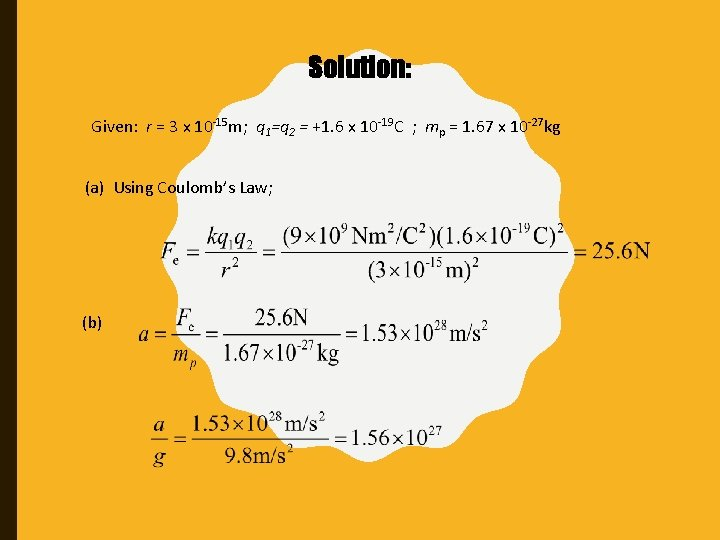Solution: Given: r = 3 x 10 -15 m; q 1=q 2 = +1. 6 x 10 -19 C ; mp = 1. 67 x 10 -27 kg (a) Using Coulomb’s Law; (b)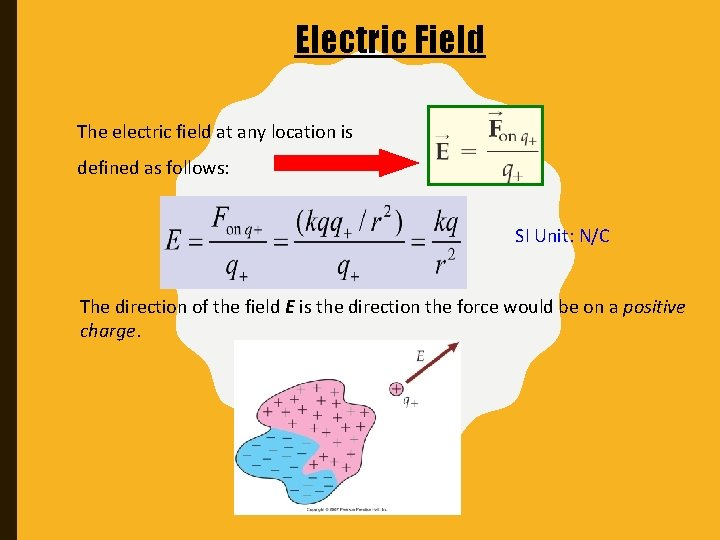Electric Field The electric field at any location is defined as follows: SI Unit: N/C The direction of the field E is the direction the force would be on a positive charge.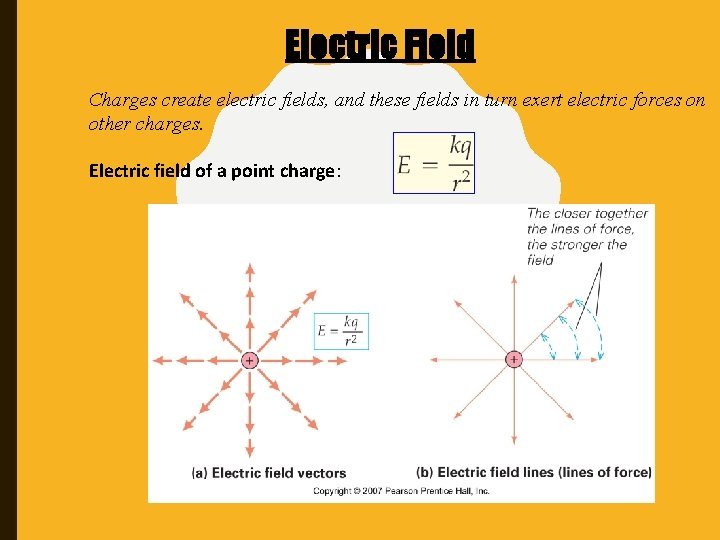Electric Field Charges create electric fields, and these fields in turn exert electric forces on other charges. Electric field of a point charge: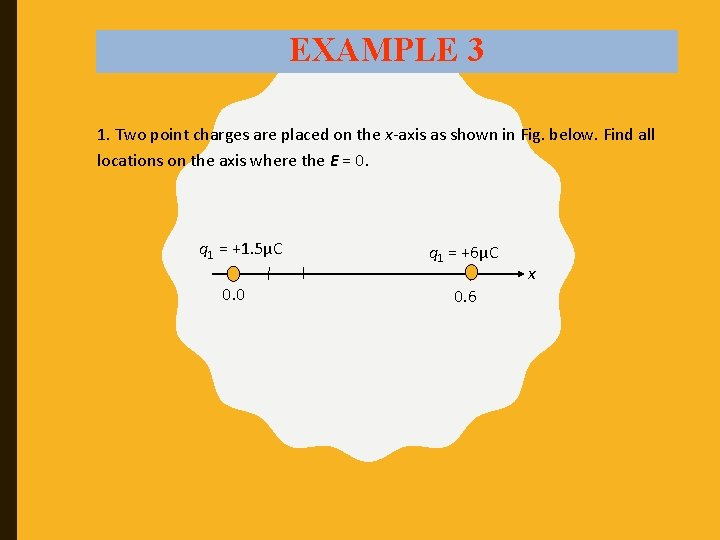EXAMPLE 3 1. Two point charges are placed on the x-axis as shown in Fig. below. Find all locations on the axis where the E = 0. q 1 = +1. 5μC 0. 0 q 1 = +6μC 0. 6 x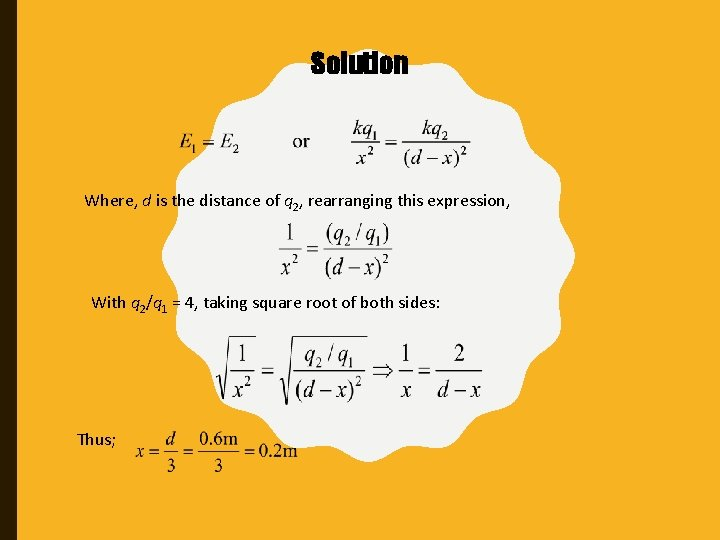Solution Where, d is the distance of q 2, rearranging this expression, With q 2/q 1 = 4, taking square root of both sides: Thus;Electric Field Electric field lines due to very large parallel plates: Q – magnitude of total charge on one of the plates; A – area of one plate.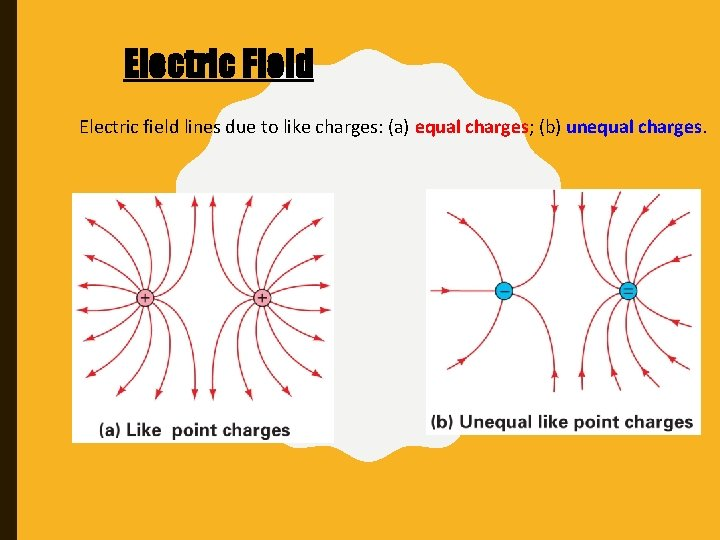Electric Field Electric field lines due to like charges: (a) equal charges; (b) unequal charges.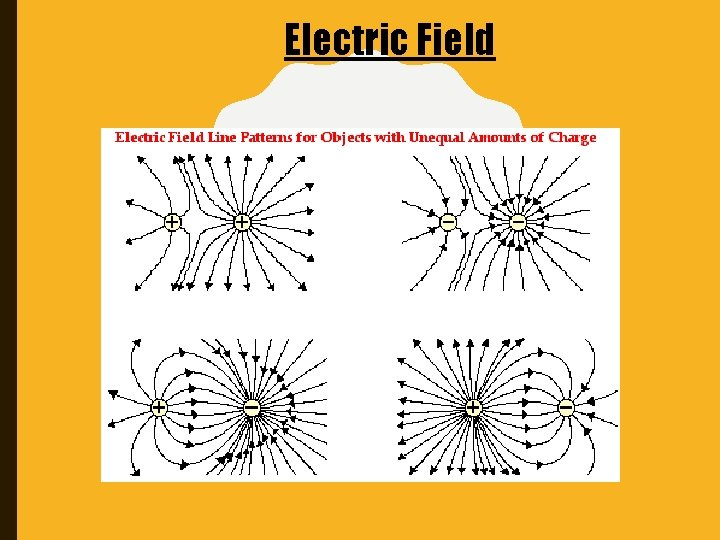Electric FieldConductors and Electric Fields Electric charges are free to move within a conductor; therefore, there cannot be a static field within the conductor: The electric field is zero inside a charged conductor. Excess charges on a conductor will repel each other, and will wind up being as far apart as possible. Any excess charge on an isolated conductor resides entirely on the surface of the conductor.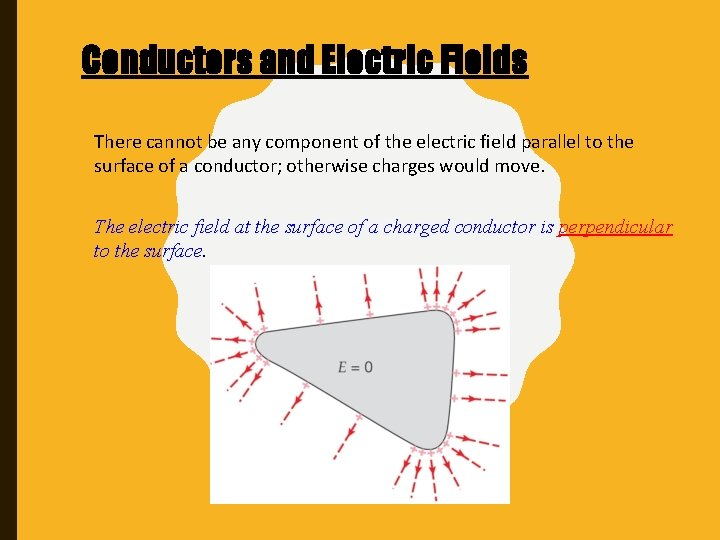Conductors and Electric Fields There cannot be any component of the electric field parallel to the surface of a conductor; otherwise charges would move. The electric field at the surface of a charged conductor is perpendicular to the surface.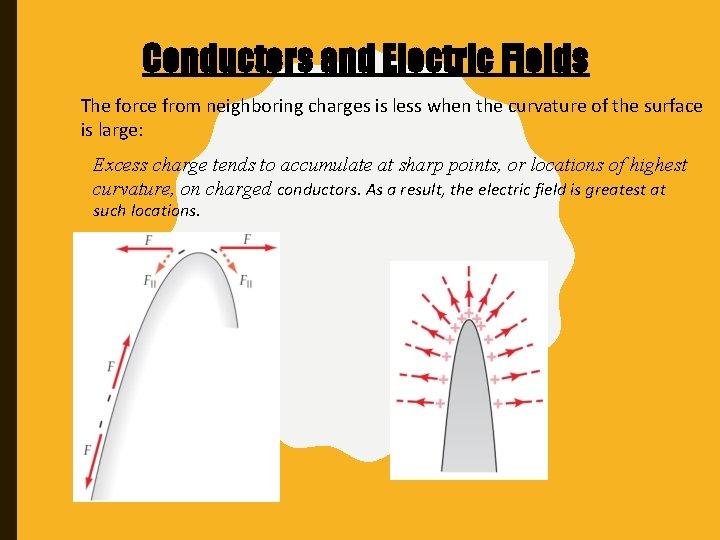Conductors and Electric Fields The force from neighboring charges is less when the curvature of the surface is large: Excess charge tends to accumulate at sharp points, or locations of highest curvature, on charged conductors. As a result, the electric field is greatest at such locations.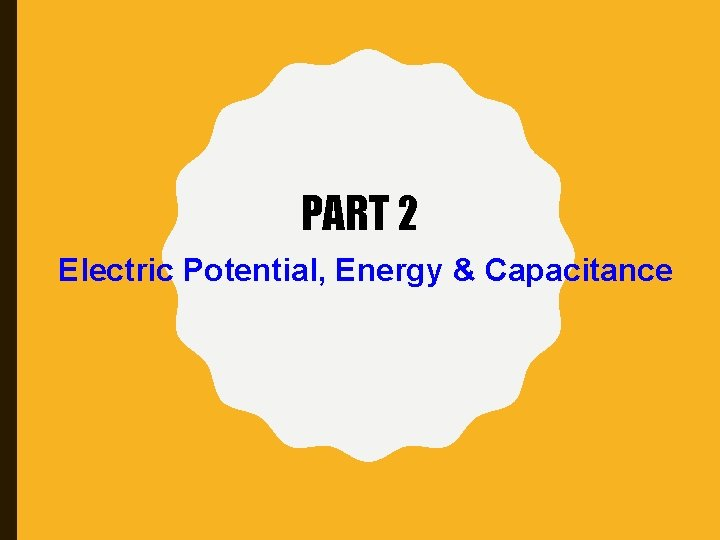PART 2 Electric Potential, Energy & Capacitance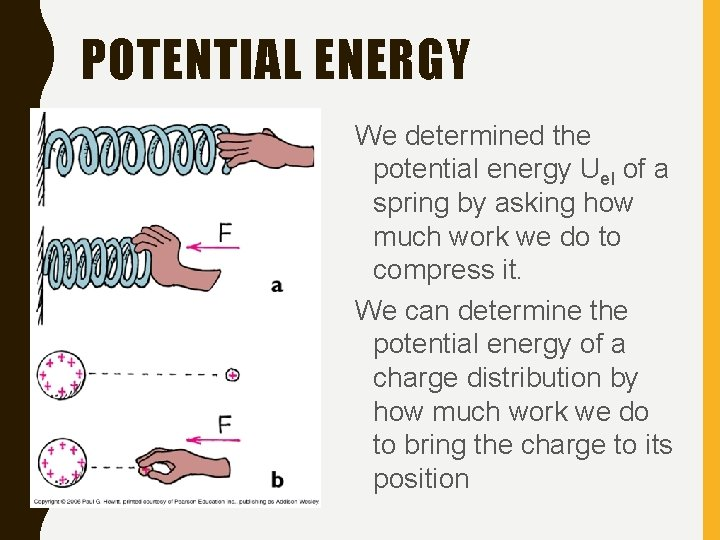POTENTIAL ENERGY We determined the potential energy Uel of a spring by asking how much work we do to compress it. We can determine the potential energy of a charge distribution by how much work we do to bring the charge to its position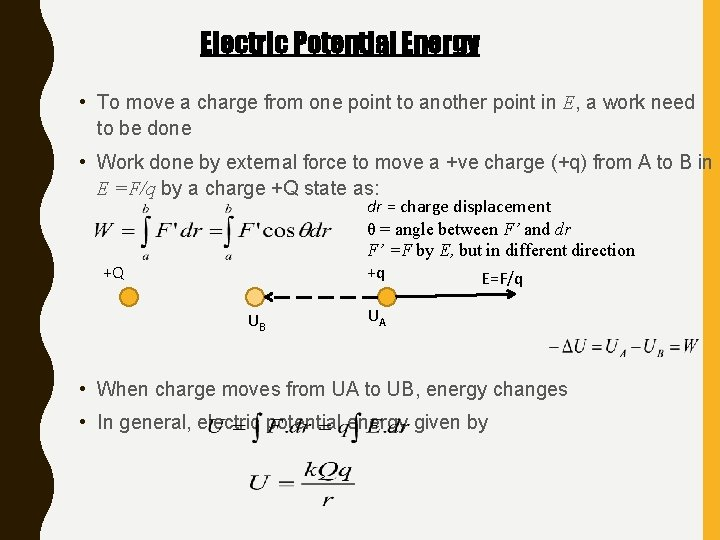Electric Potential Energy • To move a charge from one point to another point in E, a work need to be done • Work done by external force to move a +ve charge (+q) from A to B in E =F/q by a charge +Q state as: dr = charge displacement θ = angle between F’ and dr F’ =F by E, but in different direction +q E=F/q +Q UB UA • When charge moves from UA to UB, energy changes • In general, electric potential energy given byElectric Potential • Definition: Electric potential energy per unit charge • Unit: Volt or JC-1 • For a number of Q 1, Q 2 and Q 3 at distance r 1, r 2 and r 3 from point P +Q 1 r 1 P r 2 +Q 2 r 3 +Q 3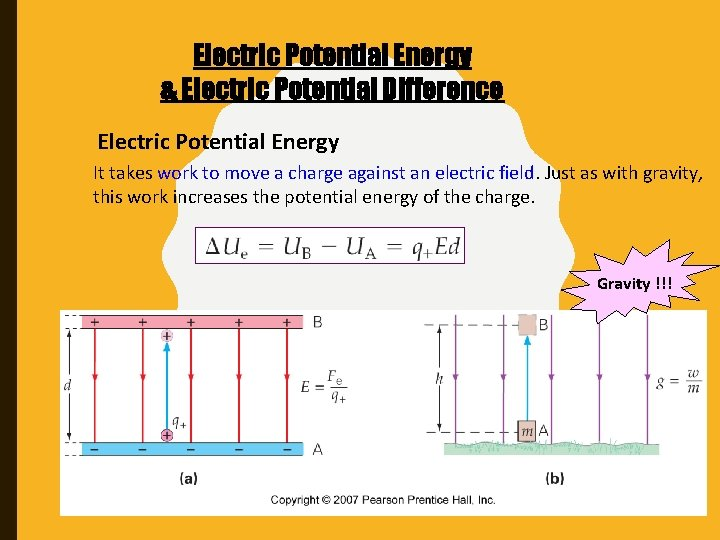Electric Potential Energy & Electric Potential Difference Electric Potential Energy It takes work to move a charge against an electric field. Just as with gravity, this work increases the potential energy of the charge. Gravity !!!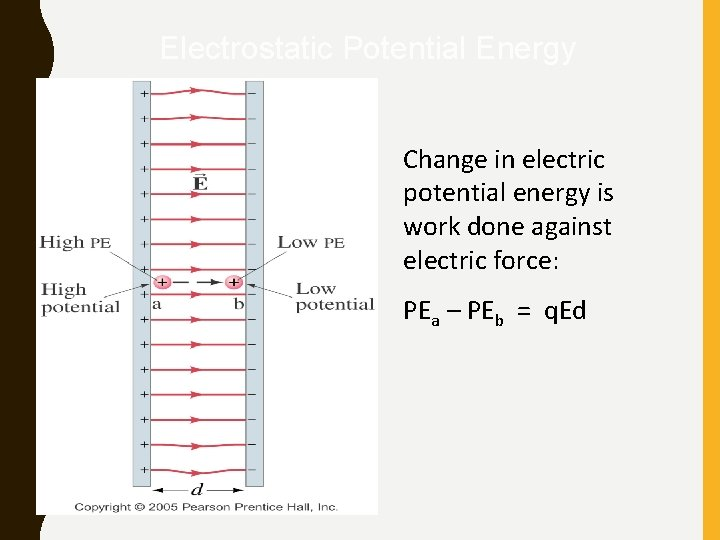Electrostatic Potential Energy Change in electric potential energy is work done against electric force: PEa – PEb = q. EdElectric Potential Energy & Electric Potential Difference Just as with the electric field, it is convenient to define a quantity that is the electric potential energy per unit charge. This is called the electric potential. Electric potential difference SI unit of electric potential: Joule/Coulomb or the volt, V.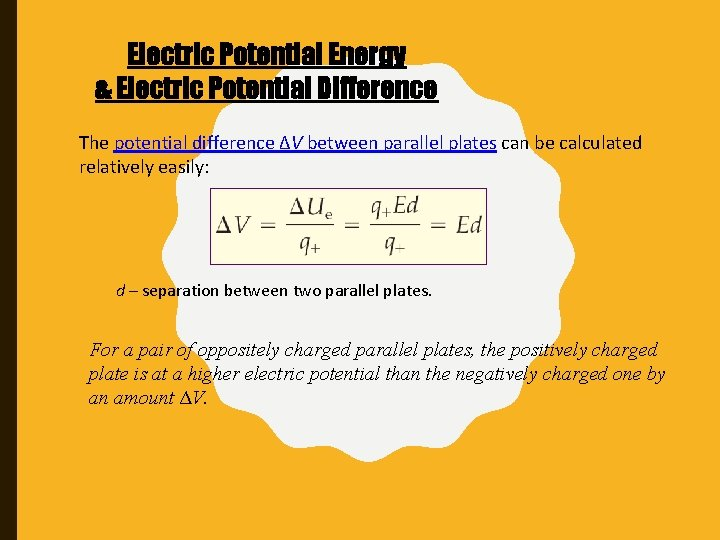Electric Potential Energy & Electric Potential Difference The potential difference ∆V between parallel plates can be calculated relatively easily: d – separation between two parallel plates. For a pair of oppositely charged parallel plates, the positively charged plate is at a higher electric potential than the negatively charged one by an amount ΔV.Electric Potential Energy & Electric Potential Difference As with potential energy, only changes in the electric potential can be defined. The choice of V = 0 is arbitrary. ∆V is independent of reference point !!!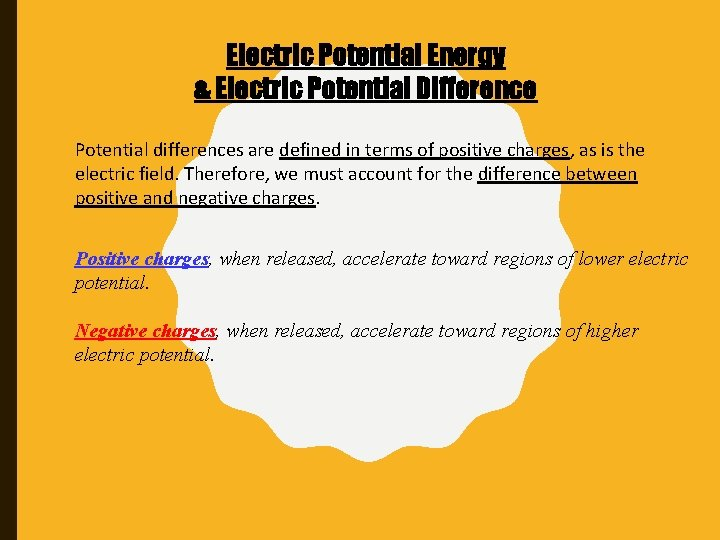Electric Potential Energy & Electric Potential Difference Potential differences are defined in terms of positive charges, as is the electric field. Therefore, we must account for the difference between positive and negative charges. Positive charges, when released, accelerate toward regions of lower electric potential. Negative charges, when released, accelerate toward regions of higher electric potential.EXAMPLE 4 Imagine moving a proton from negative plate to the positive plate of the parallel-plate arrangement. The plates are 1. 5 cm apart, and the field is uniform with a magnitude of 1500 N/C. (a) What is the change in the proton’s electric potential energy? (b) What is the electric potential difference (voltage) between the plates? (c) If the proton is released from rest at the positive plate, what is the speed will it have just before it hits the negative plate?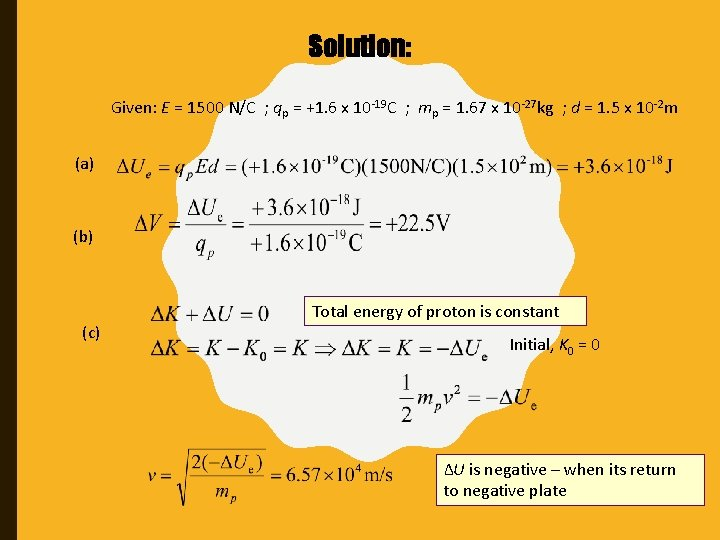Solution: Given: E = 1500 N/C ; qp = +1. 6 x 10 -19 C ; mp = 1. 67 x 10 -27 kg ; d = 1. 5 x 10 -2 m (a) (b) (c) Total energy of proton is constant Initial, K 0 = 0 ∆U is negative – when its return to negative plate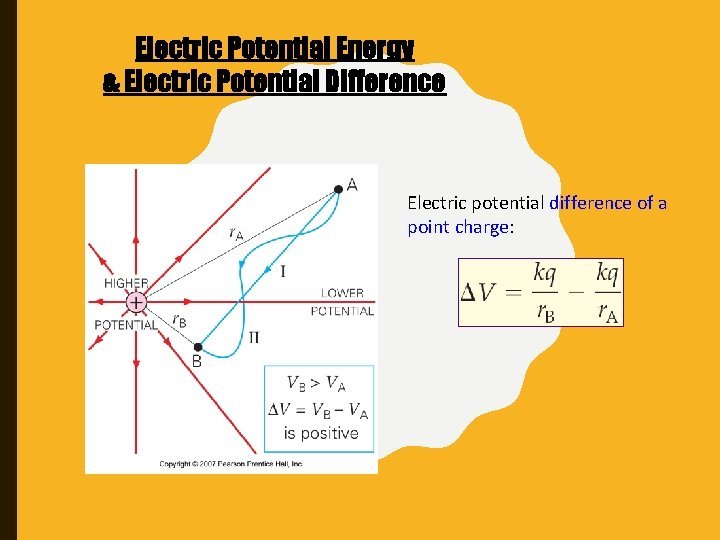Electric Potential Energy & Electric Potential Difference Electric potential difference of a point charge: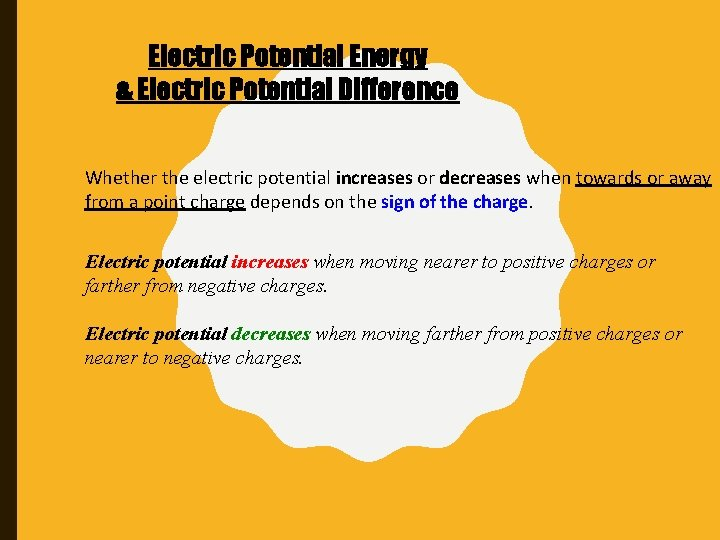Electric Potential Energy & Electric Potential Difference Whether the electric potential increases or decreases when towards or away from a point charge depends on the sign of the charge. Electric potential increases when moving nearer to positive charges or farther from negative charges. Electric potential decreases when moving farther from positive charges or nearer to negative charges.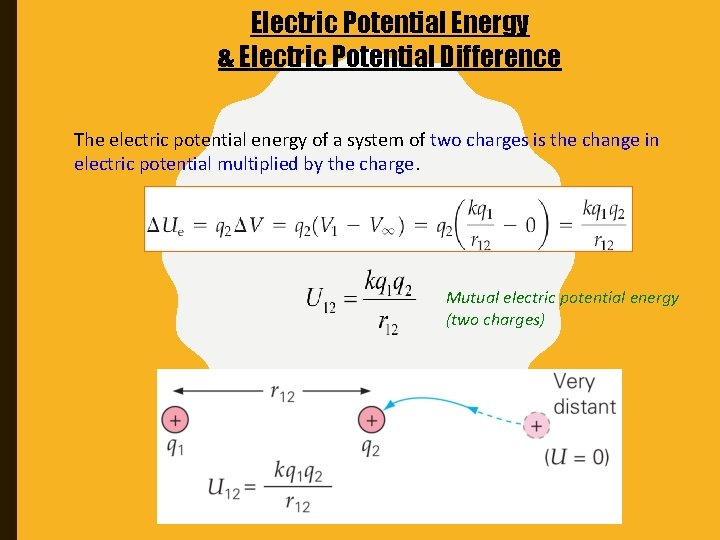Electric Potential Energy & Electric Potential Difference The electric potential energy of a system of two charges is the change in electric potential multiplied by the charge. Mutual electric potential energy (two charges)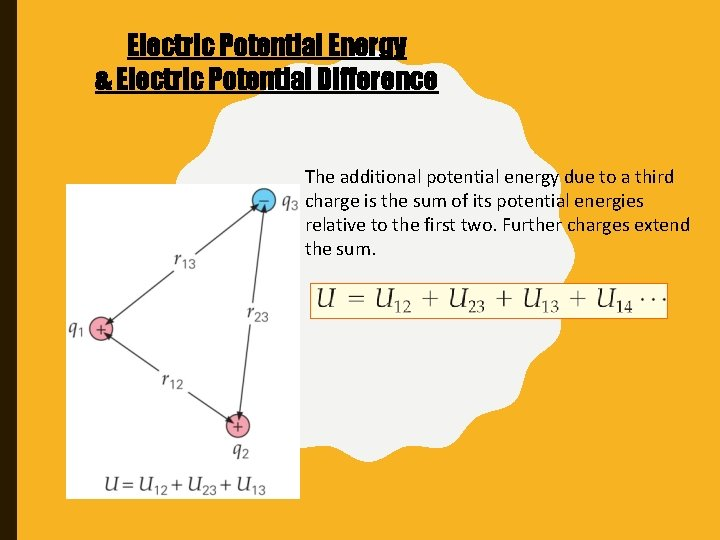Electric Potential Energy & Electric Potential Difference The additional potential energy due to a third charge is the sum of its potential energies relative to the first two. Further charges extend the sum.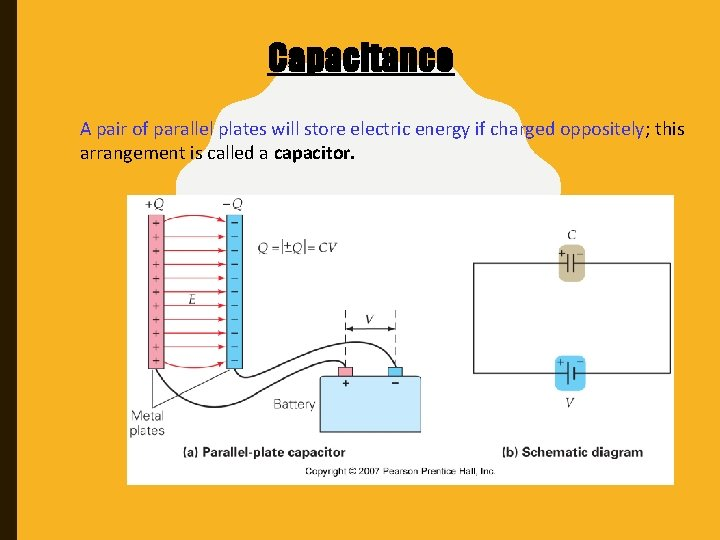Capacitance A pair of parallel plates will store electric energy if charged oppositely; this arrangement is called a capacitor.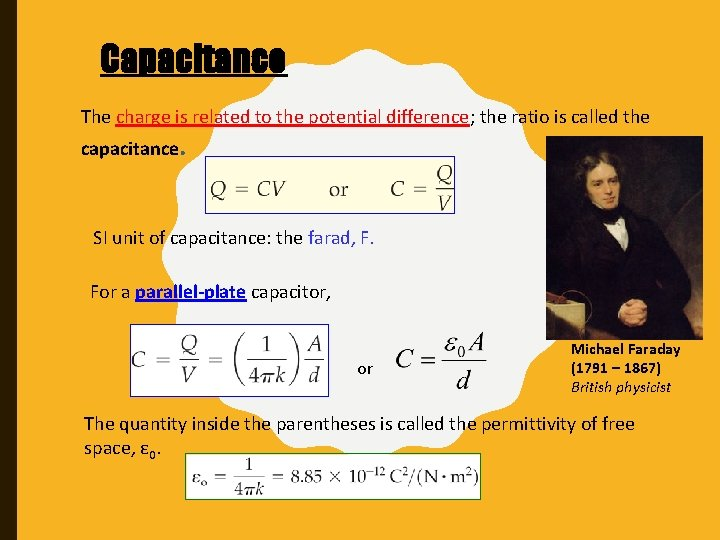Capacitance The charge is related to the potential difference; the ratio is called the capacitance. SI unit of capacitance: the farad, F. For a parallel-plate capacitor, or Michael Faraday (1791 – 1867) British physicist The quantity inside the parentheses is called the permittivity of free space, ε 0.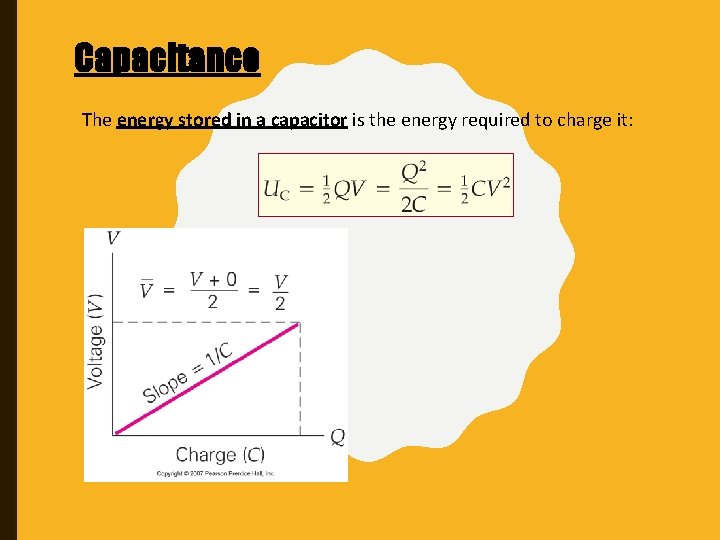Capacitance The energy stored in a capacitor is the energy required to charge it: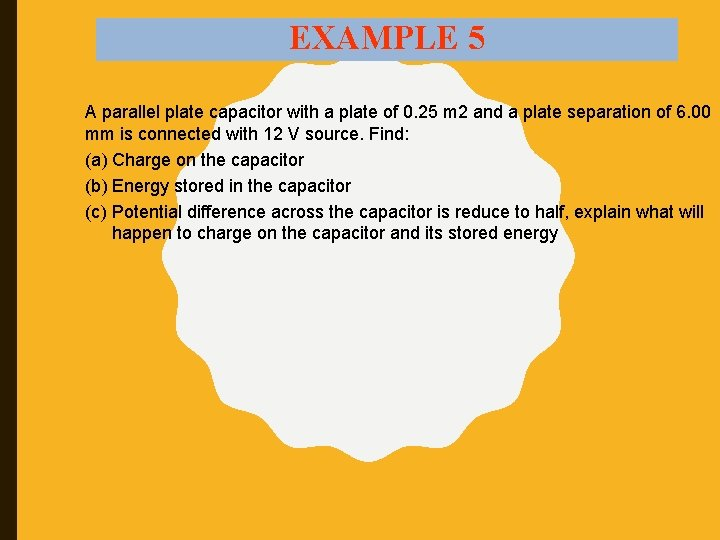EXAMPLE 5 A parallel plate capacitor with a plate of 0. 25 m 2 and a plate separation of 6. 00 mm is connected with 12 V source. Find: (a) Charge on the capacitor (b) Energy stored in the capacitor (c) Potential difference across the capacitor is reduce to half, explain what will happen to charge on the capacitor and its stored energySolution: (a) (b) (c) Since Q = CV, it half. Since UC = ½ CV 2, it doubles.Dielectrics A dielectric, or electrical insulator, is a substance that is highly resistant to the flow of an electric current. Although a vacuum is also an excellent dielectric, the following discussion applies primarily to physical substances. The use of a dielectric in a capacitor presents several advantages. The simplest of these is that the conducting plates can be placed very close to one another without risk of contact. Also, if subjected to a very high electric field, any substance will ionize and become a conductor.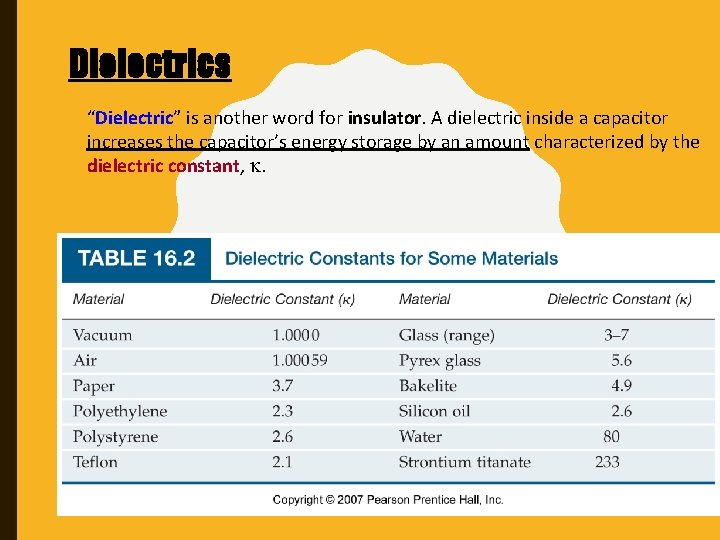Dielectrics “Dielectric” is another word for insulator. A dielectric inside a capacitor increases the capacitor’s energy storage by an amount characterized by the dielectric constant, .Dielectrics A dielectric in an electric field becomes polarized; this allows it to sustain a larger electric field for the same potential difference. The net effect: E and V <<, Stored charge remains the same - Capacitance increase.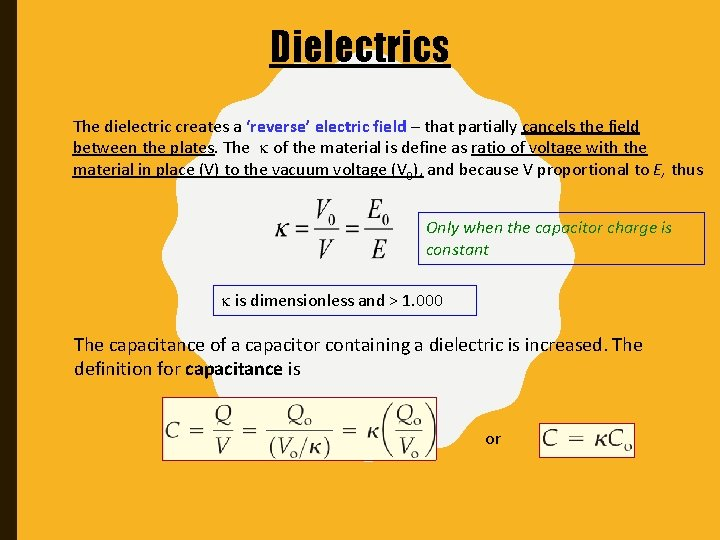Dielectrics The dielectric creates a ‘reverse’ electric field – that partially cancels the field between the plates. The of the material is define as ratio of voltage with the material in place (V) to the vacuum voltage (V 0), and because V proportional to E, thus Only when the capacitor charge is constant is dimensionless and > 1. 000 The capacitance of a capacitor containing a dielectric is increased. The definition for capacitance is orDielectrics Inserting a dielectric into a capacitor while either the voltage or the charge is held constant has the same effect – the ratio of charge to voltage increases. Voltage drops Stored energy decrease Charge on a plates increase More energy stored in capacitor